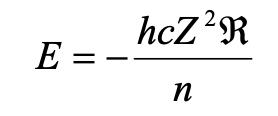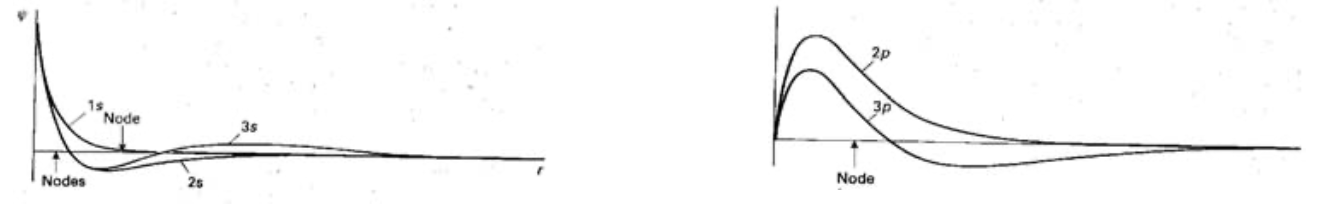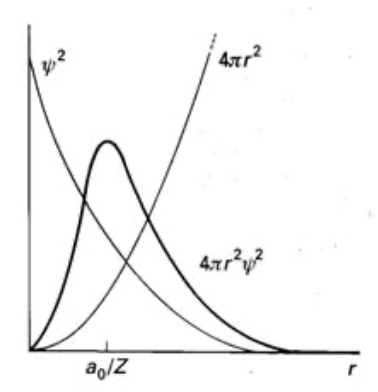# Atomic Structure

Some very basic notes on Atomic Structure and Periodicity.

# ATOMIC STRUCTURE NOTES

## Hydrogenic Atoms

### Orbital Angular Momentum & Electron Spin

#### Quantum Numbers

Principal Quantum Number - Quantized Energy

Orbital Angular Momentum, l - Quantized Orbital Angular Momentum

Magnetic Quantum Number, ml - Quantized Orientation of angular momentum.

The allowed energies are determined by n, and for a hydrogenic atom of atomic number Z:The zero energy is when electron and nucleus are widely separated. 1/n2 relationship for E indicates that the energy levels converge at high (less negative) energies. Zero energy = ionisation.

#### Electron Spin -

2 more Quantum Numbers are needed to define the state of an electron (spin).

s = spin angular momentum and always has a value of 1/2.

ms = spin magnetic quantum number, and is either +1/2 or -1/2.

These Quantum Numbers specify the orientation of the spin with respect to a chosen axis.

Spin-up and Spin-down (α and β).

### Shapes and Energies of Atomic Orbitals

#### Radial Shapes of Hydrogenic Orbitals

A Hydrogenic orbital can be written as the product of a function R of the radius and a function Y of the angular coordinates. R determines variation with distance. Y expresses the shape of the orbital.

Where the radial wavefunction passes through zero is a radial node. Where the angular wavefunction passes thru zero is an angular node or nodal plane.

1s (n=1, l=0, ml=0) decays exponentially with distance from the nucleus and never passes thru zero.

An orbital with Quantum Numbers n and l in general has n-l-1 radial nodes. Hence 2s (n=2, l=0 ml=0) passes thru zero once (one radial node).## Multi-electron Atoms

### Penetration & Screening

#### Shielding

An electron is assumed to experience an effective nuclear charge (Zeff) dependent on radius (location), n and l (differing radial distribution functions). The reduction of the true nuclear charge to the effective nuclear charge is called shielding. Zeff = Z - σ.

The closer to the nucleus that an electron can penetrate in an atom, the closer the value of Zeff to Z, because the electron is repelled less by other electrons. For example, a 2s electron can penetrate, while a 2p electron is not as effective at this because it has a nodal plane through the nucleus. Therefore, it is more shielded from the nucleus by the electrons of the core. We can conclude that a 2s electron has lower energy (more bound) than a 2p, so for Lithium the ground state configuration must be 1s22s1. It usually follows that: ns > np > nd > nf(for 1s orbital)

In general, the distance at which the electron is most likely to be found decreases with nuclear charge. The distance also increases as n increases since the higher the energy, the more likely the electron is to be further away. Maximum (most likely) position occurs at a0/Z.Although 2p is on average closer, the 2s has a higher probability of being close to the nucleus because of the inner maximum.

## Periodicity

Metallic Radius = half the distance between nuclei of neighbouring atoms.

Covalent Radius = half the inter-nuclear separation of neighbouring atoms of the same element.

General Periodic Trends - descending a group atomic radii increase, and with s & p blocks they decrease from left to right across period.

Period 6 is different, due to lanthanide contraction. The 4f orbitals are being occupied by the lanthanides, and these have poor shielding properties. The repulsions being added on crossing the f-block fail to compensate for the increasing nuclear charge, so all the electrons are drawn inwards (Zeff increases).

Similar reasoning behind the small increase in radius from Al to Ga - d-block with poor shielding.

Increase in radius of an anion is due to increased electron-electron repulsions. Smaller radius of cations is due to less repulsion and also that there is a less in valence electrons (leaving a more compact core).

### Ionization Energy

Follows pattern of Zeff. Correlate with atomic radii - small radius = high EI.

### Electron Affinity

Electron-gain enthalpy - ∆egH is the standard enthalpy change per mole of atoms when a gas-phase atom gains an electron. Can be exothermic or endothermic.

A(g) + e-(g) --> A-(g)

Ea = E(A,g) - E(A-,g)

A positive electron affinity indicates that the ion has a lower (more -ve), and so more favourable energy than the neutral atom. Its value is determined by the energy of the lowest unfilled orbital of the ground-state atom. An element has a high electron affinity if the additional electron can enter a shell where it experiences a strong effective nuclear charge. This is the case for elements in the top right of the periodic table.